# Python Program to perform cross join in Pandas

In Pandas, there are parameters to perform left, right, inner or outer merge and join on two DataFrames or Series. However there’s no possibility as of now to perform a cross join to merge or join two methods using `how="cross"` parameter.

Cross Join :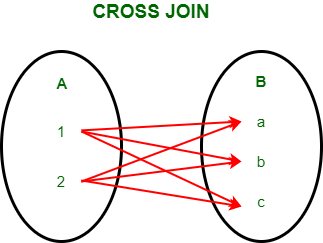Example 1:

The above example is proven as follows

 `# importing pandas module ` `import` `pandas as pd  ` `  `  `# Define a dictionary with column A ` `data1 ``=` `{``'A'``: [``1``, ``2``]}  ` `    `  `# Define another dictionary with column B ` `data2 ``=` `{``'B'``: [``'a'``, ``'b'``, ``'c'``]}   ` `  `  `# Convert the dictionary into DataFrame   ` `df ``=` `pd.DataFrame(data1, index ``=``[``0``, ``1``]) ` `  `  `# Convert the dictionary into DataFrame   ` `df1 ``=` `pd.DataFrame(data2, index ``=``[``2``, ``3``, ``4``])  ` ` `  `# Now to perform cross join, we will create ` `# a key column in both the DataFrames to  ` `# merge on that key. ` `df[``'key'``] ``=` `1` `df1[``'key'``] ``=` `1` ` `  `# to obtain the cross join we will merge  ` `# on the key and drop it. ` `result ``=` `pd.merge(df, df1, on ``=``'key'``).drop(``"key"``, ``1``) ` ` `  `result `

DataFrame 1: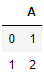DataFrame 2 :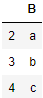Output :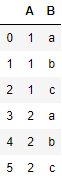Example 2:

Cross join on two DataFrames for user and product.

 `# importing pandas module ` `import` `pandas as pd  ` `  `  `# Define a dictionary containing user ID ` `data1 ``=` `{``'Name'``: [``"Rebecca"``, ``"Maryam"``, ``"Anita"``], ` `        ``'UserID'``: [``1``, ``2``, ``3``]}  ` `    `  `# Define a dictionary containing product ID  ` `data2 ``=` `{``'ProductID'``: [``'P1'``, ``'P2'``, ``'P3'``, ``'P4'``]}  ` `  `  `# Convert the dictionary into DataFrame   ` `df ``=` `pd.DataFrame(data1, index ``=``[``0``, ``1``, ``2``]) ` `  `  `# Convert the dictionary into DataFrame   ` `df1 ``=` `pd.DataFrame(data2, index ``=``[``2``, ``3``, ``6``, ``7``])  ` ` `  `# Now to perform cross join, we will create ` `# a key column in both the DataFrames to  ` `# merge on that key. ` `df[``'key'``] ``=` `1` `df1[``'key'``] ``=` `1` ` `  `# to obtain the cross join we will merge on  ` `# the key and drop it. ` `result ``=` `pd.merge(df, df1, on ``=``'key'``).drop(``"key"``, ``1``) ` ` `  `result `

DataFrame 1: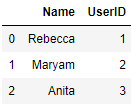DataFrame 2 :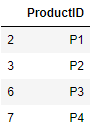Output :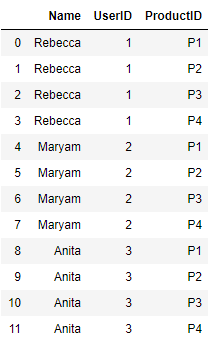Example 3:

 `# importing pandas module ` `import` `pandas as pd  ` `  `  `# Define a dictionary with two columns ` `data1 ``=` `{``'col 1'``: [``0``, ``1``], ` `        ``'col 2'``: [``2``, ``3``]}  ` `    `  `# Define another dictionary  ` `data2 ``=` `{``'col 3'``: [``5``, ``6``], ` `        ``'col 4'``: [``7``, ``8``]}   ` `  `  `# Convert the dictionary into DataFrame   ` `df ``=` `pd.DataFrame(data1, index ``=``[``0``, ``1``]) ` `  `  `# Convert the dictionary into DataFrame   ` `df1 ``=` `pd.DataFrame(data2, index ``=``[``2``, ``3``])  ` ` `  `# Now to perform cross join, we will create ` `# a key column in both the DataFrames to ` `# merge on that key. ` `df[``'key'``] ``=` `1` `df1[``'key'``] ``=` `1` ` `  `# to obtain the cross join we will merge on  ` `# the key and drop it. ` `result ``=` `pd.merge(df, df1, on ``=``'key'``).drop(``"key"``, ``1``) ` ` `  `result `

DataFrame 1: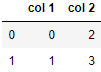DataFrame 2 :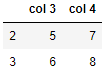Output :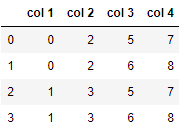My Personal Notes arrow_drop_upCheck out this Author's contributed articles.

If you like GeeksforGeeks and would like to contribute, you can also write an article using contribute.geeksforgeeks.org or mail your article to contribute@geeksforgeeks.org. See your article appearing on the GeeksforGeeks main page and help other Geeks.

Please Improve this article if you find anything incorrect by clicking on the "Improve Article" button below.

Article Tags :

Be the First to upvote.

Please write to us at contribute@geeksforgeeks.org to report any issue with the above content.